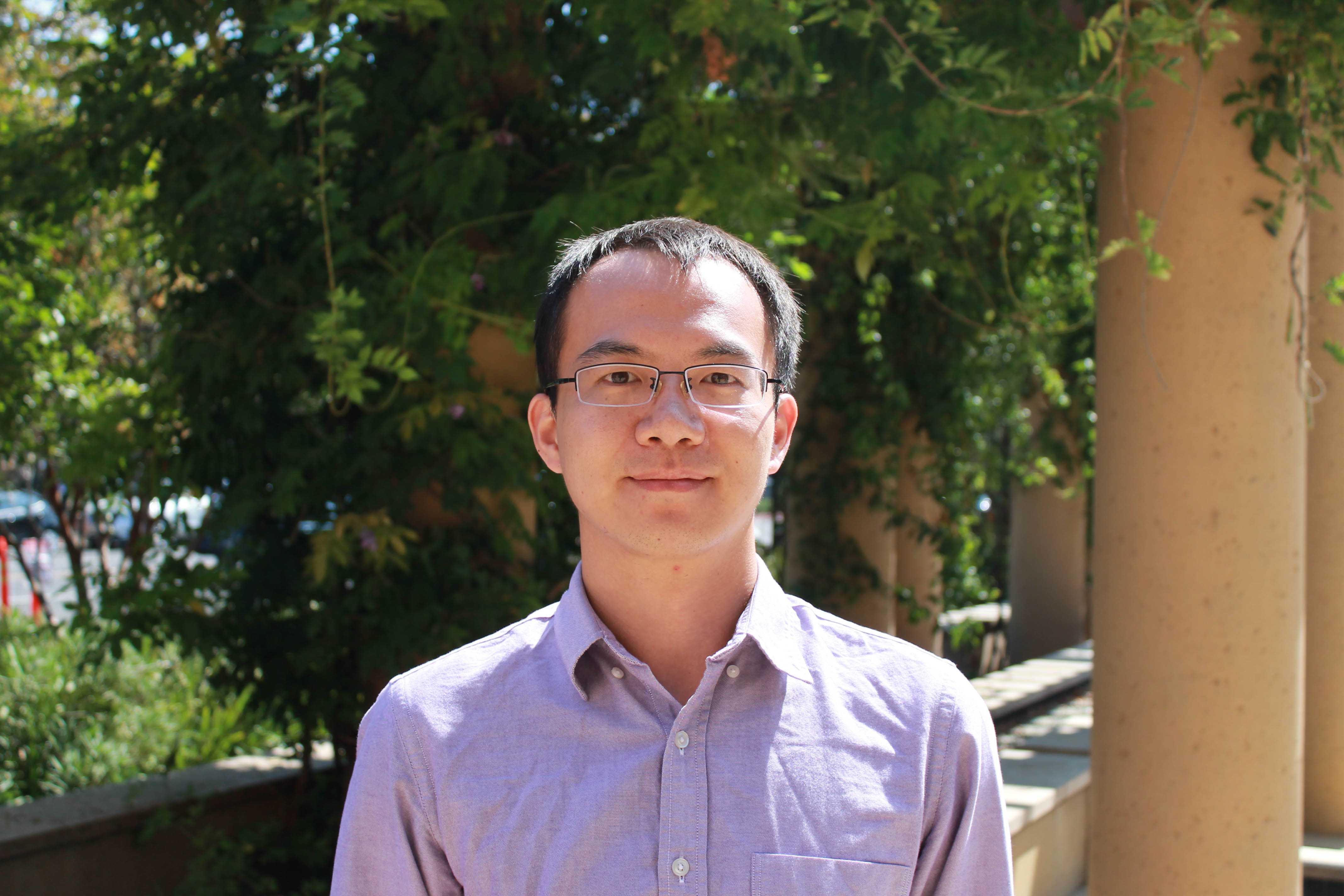# Chao ChenPh.D. Candidate Institute for Computational and Mathematical Engineering (ICME) Standford University M.S. in ICME, Stanford University, U.S., 2014 B.S. in Mathematics, Nankai University, China, 2012 Adviser: Eric Darve Dissertation topic: “Parallel hierarchical solvers for general sparse linear systems with applications to ice sheet modeling” Contact: cchen10@stanford.edu 452 Panama St Building 520, Room 225, Stanford, CA 94305 Software: https://github.com/Charles-Chao-Chen

I am an applied mathematician who uses computational tools to solve partial differential equations. The modeling of natural phenomena may lead to large-scale numerical simulations that require significant processing units on supercomputers. Within these simulations, solving the discretized linear systems is typically the most time-consuming component.

I work on developing new algorithms using hierarchical matrices. These methods can be faster than direct methods based on Gaussian elimination and more robust than iterative methods, such as incomplete LU factorizations.

I use parallel computing to implement new algorithms efficiently on large-scale supercomputers. I have used both the classical Message Passing Interface (MPI) and modern task-based runtime systems, and my codes run on some of the largest/fastest supercomputers in the world.

My research interests are

• Parallel linear solvers/pre-conditioners using hierarchical matrices

• Parallel computing, e.g., distributed-memory computing

• Fast numerical methods, e.g., the fast multipole method